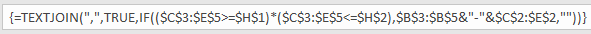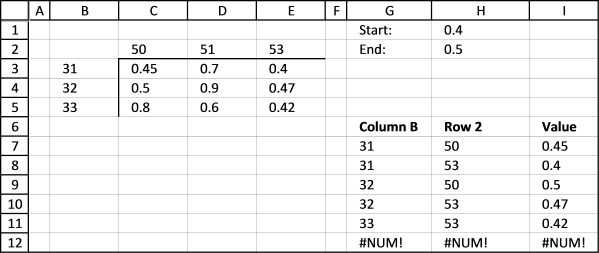Author: Oscar Cronquist Article last updated on November 27, 2017

The excel 2016 formula lets you concatenate all values in one cell and it works only in excel 2016 because of the TEXTJOIN function. You only need one formula and it is small and easy to understand.

The version that works with all excel versions lets you put values in a cell each however the formulas are a little bit more complicated.

I have this table where there are two dependent variables.
tableRow 2 contains variable and Column B contains another variable
The combination of 32 with 51 gives me 0.9 or cell D4
I need to report which 2 and B combinations give me values between 0.4 and 0.5. In this case, it would be 31-53 and 32-50.
I could rearrange this to be in 3 columns with the results on the last column and use something like below (The equation does not represent the example I gave.)
IFERROR(INDEX(array, SMALL(IF((min=data), ROW(\$B\$2:\$B\$10)-1), ROW(A3)), COLUMN(A3)), " ")
This works but my excel table would have too many rows and the initial data set comes the other way so it would take some time to convert it. The original matrix is more compact.
I am trying to convert this equation but I am having trouble matching it to an array instead to just one column.

The following array formula returns multiple values from a cross reference table if they meet specific critera.

Array formula in cell H3:

=TEXTJOIN(",", TRUE, IF((\$C\$3:\$E\$5>=\$H\$1)*(\$C\$3:\$E\$5<=\$H\$2), \$B\$3:\$B\$5&"-"&\$C\$2:\$E\$2,""))

If you prefer having the values in a cell each instead of concatenated values in one cell, go to this part of this article.

#### How to build an array formula

1. Select cell H3
2. Type the formula above in formula bar3. Press and hold CTRL + SHIFT key
4. Press Enter

If you did above steps correctly excel automatically adds a beginning and ending curly bracket to the formula, like this:Don't enter these characters yourself.

#### Explaining array formula in cell G3

Note, TEXTJOIN function works only in Excel 2016. If you have an earlier version of excel, use this formula.

Step 1 - Check if values in cell range C3:E5 are larger than H1 and smaller than H2

IF((\$C\$3:\$E\$5>=\$H\$1)*(\$C\$3:\$E\$5<=\$H\$2), \$B\$3:\$B\$5&"-"&\$C\$2:\$E\$2,"")

becomes

IF({0,0,1;1,0,0;0,0,0}, \$B\$3:\$B\$5&"-"&\$C\$2:\$E\$2,"")

Step 2 - If TRUE concatenate corresponding values in row 2 and column B

IF({0,0,1;1,0,0;0,0,0}, \$B\$3:\$B\$5&"-"&\$C\$2:\$E\$2,"")

becomes

IF({0,0,1;1,0,0;0,0,0}, {"31-50","31-51","31-53";"32-50","32-51","32-53";"33-50","33-51","33-53"},"")

and returns

{"","","31-53";"32-50","","";"","",""}

Step 3 - Concatenate values using TEXTJOIN function

TEXTJOIN(",",TRUE,{"","","31-53";"32-50","","";"","",""})

returns

31-53. 32-50 in cell H3.

Link to two-way lookups in an index table:
Looking up data in a cross reference table

Reverse two-way lookup in a cross reference table.xlsx

### Reverse two-way lookups in a cross reference table

This formula is for excel versions that don't have the TEXTJOIN function or if you prefer having the values in a cell each instead of concatenated values in one cell.Array formula in cell G7:

=INDEX(\$B\$3:\$B\$5, SMALL(IF((\$C\$3:\$E\$5<=\$H\$2)*(\$C\$3:\$E\$5>=\$H\$1), MATCH(ROW(\$B\$3:\$B\$5), ROW(\$B\$3:\$B\$5)), ""), ROW(A1)))

Array formula in cell H7:

=INDEX(\$C\$2:\$E\$2, SMALL(IF((\$C\$3:\$E\$5<=\$H\$2)*(\$C\$3:\$E\$5>=\$H\$1)*(G7=\$B\$3:\$B\$5) ,MATCH(COLUMN(\$C\$2:\$E\$2), COLUMN(\$C\$2:\$E\$2)), ""), COUNTIF(\$G\$6:G7,G7)))

Array formula in cell I7:

=INDEX(\$C\$3:\$E\$5, MATCH(G7,\$B\$3:\$B\$5, 0), MATCH(H7, \$C\$2:\$E\$2, 0))

How to enter an array formula

#### Explaining formula in cell I7

Reverse-two-way-lookup-in-a-cross-reference-tablev3.xlsx

### Reverse two-way lookup in a cross reference tableThe following array formulas returns a single value from a cross reference table.

Array formula in cell E15:

=INDEX(\$A\$3:\$A\$12, MIN(IF(B3:K12=E14, MATCH(ROW(B3:K12), ROW(B3:K12)), "")))

Array formula in cell E16:

=INDEX(\$B\$2:\$K\$2, MIN(IF(B3:K12=E14, MATCH(COLUMN(B3:K12), COLUMN(B3:K12)), "")))

How to enter an array formula

#### Explaining array formula in cell E15

Step 1 - Check what values in cell range equals value in E14 and return smallest row

MIN(IF(B3:K12=E14, MATCH(ROW(B3:K12), ROW(B3:K12)), ""))

returns 5.

Step 2 - Return corresponding header value

=INDEX(\$A\$3:\$A\$12, 5)

returns H.

Reverse two-way lookup in a cross reference tablev2.xlsx

#### Functions in this post:

TEXTJOIN(delimiter, ignore_empty, text1, ...)
Concatenates a list or range of text strings using a delimiter

IF(logical_test, [value_if_true], [value_if_false])
Checks whether a condition is met, and returns one value if TRUE, and another value if FALSE

MATCH(lookup_value, lookup_array, [match_type])
Returns the relative position of an item in an array that matches a specified value

INDEX(array,row_num,[column_num])
Returns a value or reference of the cell at the intersection of a particular row and column, in a given range

ROW(reference)
Returns the rownumber of a reference